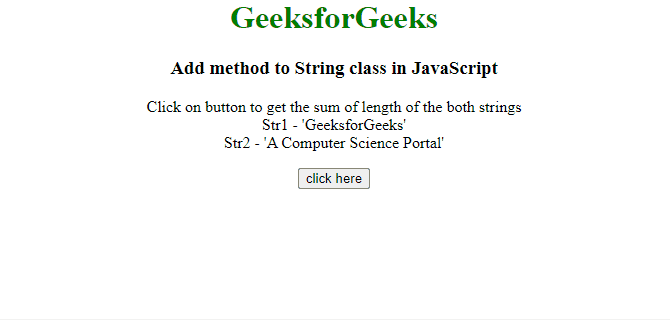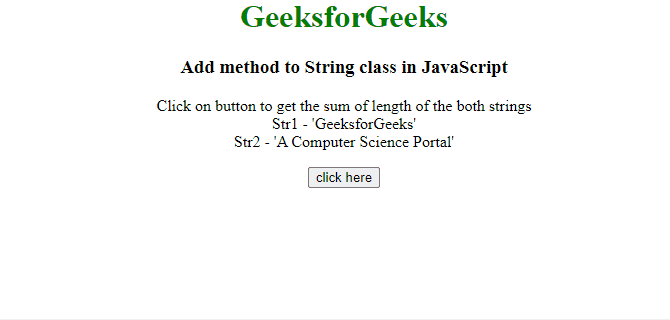GFG App
Open AppBrowser
Continue

# How to add method to String class in JavaScript ?

In this article, the task is to add a method to the String class in JavaScript. There are two approaches that are described with the proper examples:

Approach 1: Use the Object.defineProperty() method, which is used to define a new property directly to an object, or modify an existing property. It takes 3 arguments, the first is Object, the Second is propertyName, and the last is propertyDescription. In this example, the sum of the length of strings is returned.

Example: This example uses the above-explained approach.

## html

 `<``body` `style``=``"text-align: center"``> ` `    ``<``h1` `style``=``"color: green"``> ` `        ``GeeksforGeeks ` `    `` ` `    ``<``h3``> ` `        ``Add method to String class in JavaScript ` `    `` ` ` `  `    ``<``p` `id``=``"GFG_UP"``> ` ` `  `    ``<``button` `onClick``=``"GFG_Fun()"``> ` `        ``click here ` `    `` ` ` `  `    ``<``p` `id``=``"GFG_DOWN"``> ` ` `  `    ``<``script``> ` `        ``var up = document.getElementById('GFG_UP'); ` `        ``var down = document.getElementById('GFG_DOWN'); ` `         `  `        ``var str1 = "GeeksforGeeks"; ` `        ``var str2 = "A Computer Science Portal"; ` `         `  `        ``up.innerHTML = "Click on button to get the " ` `                    ``+ "sum of length of the both " ` `                    ``+ "strings<``br``>Str1 - '" + str1 ` `                    ``+ "'<``br``>Str2 - '" + str2 + "'"; ` `         `  `        ``Object.defineProperty(String.prototype, ` `        ``'SumOfLength', { ` `            ``value: function(param) { ` `                ``return this.length + param.length; ` `            ``} ` `        ``}); ` `         `  `        ``function GFG_Fun() { ` `            ``const res = str1.SumOfLength(str2); ` `            ``down.innerHTML = res; ` `        ``} ` `    `` ` ``

Output:Approach 2: Use String.prototype.propertyName to add a new method to the String class. Define a method that takes arguments passed by the object and performs the desired operation. In this example, the sum of the length of strings is returned.

Example: This example uses the above-explained approach.

## html

 `<``body` `style``=``"text-align: center"``> ` `    ``<``h1` `style``=``"color: green"``>GeeksforGeeks ` `    ``<``h3``> ` `        ``Add method to String ` `        ``class in JavaScript ` `    `` ` ` `  `    ``<``p` `id``=``"GFG_UP"``> ` ` `  `    ``<``button` `onClick``=``"GFG_Fun()"``> ` `        ``click here ` `    `` ` ` `  `    ``<``p` `id``=``"GFG_DOWN"``> ` ` `  `    ``<``script``> ` `        ``var up = document.getElementById('GFG_UP'); ` `        ``var down = document.getElementById('GFG_DOWN'); ` `         `  `        ``var str1 = "GeeksforGeeks"; ` `        ``var str2 = "A Computer Science Portal"; ` `         `  `        ``up.innerHTML = "Click on button to get the " ` `                    ``+ "sum of length of the both " ` `                    ``+ "strings<``br``>Str1 - '" + str1 ` `                    ``+ "'<``br``>Str2 - '" + str2 + "'"; ` `         `  `        ``String.prototype.SumOfLength = function( arg ) { ` `            ``return this.length + arg.length; ` `        ``}; ` `        ``function GFG_Fun() { ` `            ``const res = str1.SumOfLength(str2); ` `            ``down.innerHTML = res; ` `        ``} ` `    `` ` ``

Output: